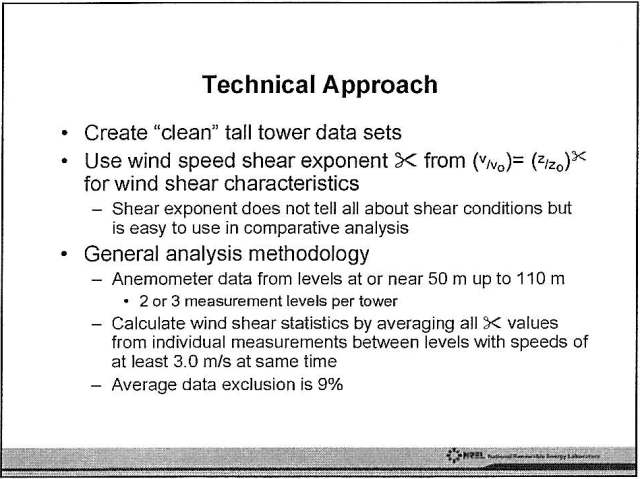## excel: alpha code to numbers

I use an annoying program that labels its output A..Z, AA..AZ, BA … rather than numerically from 1. This is annoying, as a spreadsheet won’t sort it correctly (it does A, AA, AB …). The following code will convert this code to the right numbers, assuming your alpha code is in cell B3:

`=IF(LEN(B3)=1,CODE(B3)-64,26*(CODE(B3)-64)+CODE(RIGHT(B3))-64)`

This will only work for codes of two characters or less, and is case sensitive.

## scissors, shears, geddit?!The presenter of this paper claimed that PowerPoint changed Î±, the wind shear coefficient, to âœ‚. We laughed, briefly.